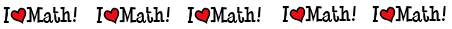# Math Review Videos

```
```
 Long Division  (13 mins. 3 sec.)Multi-Digit Multiplication (6 mins. 40 sec.)Rounding Whole Numbers  (4 mins. 50 sec.)Prime Factorization (Factor Trees) (7 mins. 14 sec.)Solving Equations -- Addition & Subtraction  (6 mins. 10 sec.)Solving Equations -- Multiplication & Division  (6 mins. 5 sec.)Adding & Subtracting Integers (5 mins. 40 sec.)Percentages (8 mins. 29 sec.)Adding & Subtracting Fractions with Like and Unlike Denominators (8 mins. 46 sec.)Multiplying Fractions (5 mins. 9 sec.)Multiplying Mixed Numbers (5 mins. 23 sec.)Dividing Fractions & Mixed Numbers (6 mins. 10 sec.)Proportions & Cross Multiplying (8 mins. 51 sec.)Rounding Fractions (6 mins. 11 sec.)Divisibility Rules & Prime Factorization (6 mins. 11 sec.)Factors & Greatest Common Factors (3 mins. 22 sec.)Reducing Fractions, Converting Improper Fractions to Mixed Numbers, & Converting Mixed Numbers to Improper Factions (5 mins. 16 sec.)Least Common Multiple & Comparing Fractions (7 mins. 15 sec.)Converting Decimals to Fractions &  Fractions to Decimals (5 mins. 22 sec.)Volume of Composed Figures

 Videos created by Mr. Coley